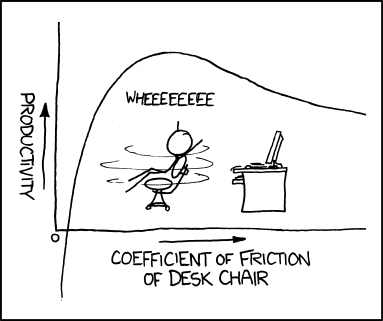# STATISTICS LEVEL 1 - BIVARIATE DATA

• Posted on: 26 May 2017
• By: louisewilson
Block:
Term:
Year:
LA Code:
Code:
Room:
Learning Area:
Levels:
6

In statistics, bivariate data is data that has two variables. The quantities from these two variables are often represented using a scatter plot. This is done so that the relationship (if any) between the variables is easily seen. For example, bivariate data on a scatter plot could be used to study the relationship between stride length and length of legs.Students need to be familiar with using the statistical enquiry cycle to investigate bivariate numerical data, which involves:

• planning and conducting an investigation using bivariate numerical data
• working with a given relationship question
• determining appropriate variables and measures
• managing sources of variation
• gathering data
• selecting and using appropriate display(s)
• communicating relationship(s) in the data in a conclusion.Achievement: Investigate bivariate numerical data using the statistical enquiry cycle.

Achievement with Merit: Investigate bivariate numerical data using the statistical enquiry cycle with justification.

Achievement with Excellence: Investigate bivariate numerical data using the statistical enquiry cycle with statistical insight.

Assessment Opportunities: